##### Trigonometry Workbook For DummiesOn scientific calculators, the –1 or x1 button means to find the reciprocal of a number. This reciprocal button allows you to find the value of a reciprocal function when you’re working with a number. But look under the 2nd functions, which are different functions or operations written above the buttons, for the inverse trig functions.

Access to these functions is usually above the original sine, cosine, and tangent buttons. Some calculators have a button labeled “2nd.” Others use alternate colors — usually yellow or green — to denote the second use of the button. And even when you find the inverse functions, you’ll notice that they’re only for the three primary trig functions.

The calculator doesn’t show any way to access cosecant, secant, or cotangent. So where are they? The following information shows how to use the three available buttons and then how to calculate the other inverses.

## Using the inverse function button on your scientific calculator

To understand this button, look at the following example: find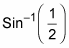in degrees by using a scientific calculator.

For this example, use the mode menu or whatever method your calculator uses to change the mode to degrees.

2. Enter the problem as given.

The following are the typical keystrokes: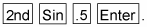The result is 30, meaning 30 degrees.

## Calculating the inverse of a reciprocal function on your scientific calculator

To determine the inverse of a reciprocal function, such as Cot1(2) or Sec1(–1), you have to change the problem back to the function’s reciprocal — one of the three basic functions — and then use the appropriate inverse button.

When changing to the function’s reciprocal, you flip the number with that function, too. For example, Cot1(2) becomes

## Working around the inverse cotangent

The other big pitfall you encounter when using a scientific calculator involves the inverse cotangent. The inverse tangent, Tan1x, has its range in QI and QIV, but Cot1x has its range in QI and QII. If you want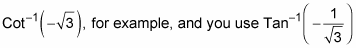and your calculator, you get an answer in the fourth quadrant. You have to be aware that this quadrant isn’t correct; you got it because you changed functions so you could use the calculator. Just use the answer from the calculator and determine the corresponding angle in QII. Here’s an example.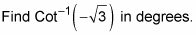1. Set the mode to degrees.

2. Change the function and value to their reciprocals.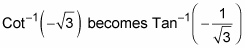3. Find the value of the inverse function by using a calculator.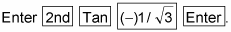On some calculators, parentheses will automatically pop up for you to enter the tangent value inside them. If they don’t, then you should insert parentheses around the fraction manually. The result is –30 degrees.

4. Find the angle in QII that has the same reference angle.

The angle in QII with a 30-degree reference angle is an angle of 150 degrees.